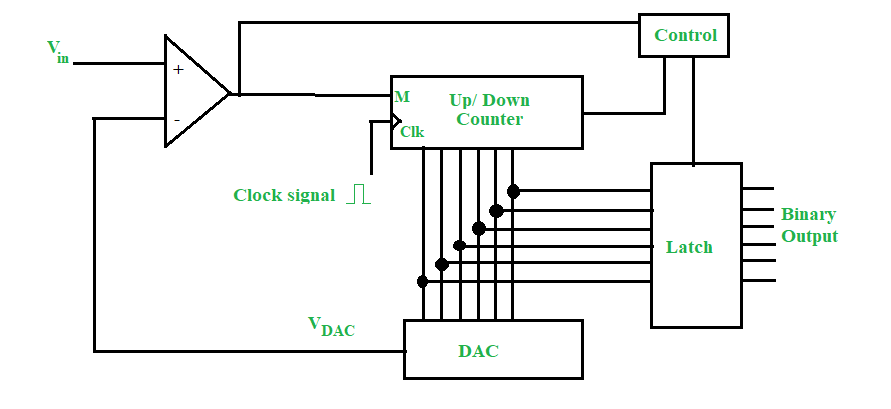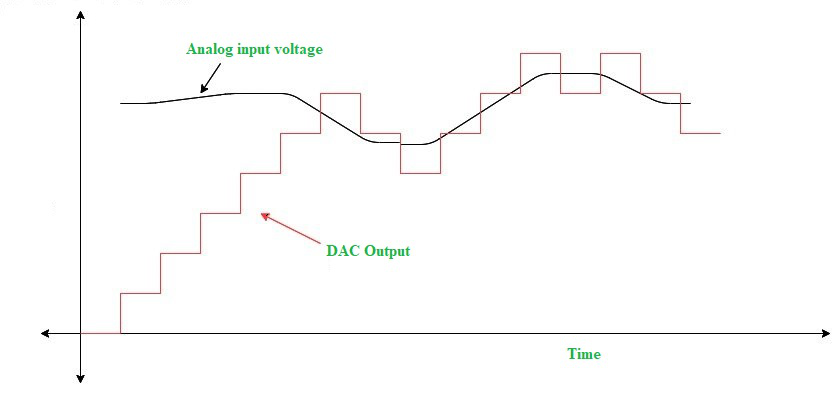• Last Updated : 04 Jul, 2022

The Counter-Type Analog-to-Digital Converter (ADC) starts conversion right from the start for each input value. This means the counter has to count from all 0s to the input value N and it takes (N-1)TC time, where Tc is the clock pulse time period. The maximum conversion time for the full-scale output range takes (2n-1)Tc seconds. This is the main drawback of counter-type ADC, besides its robustness and simplicity.

To overcome this problem of each count starting right from all 0s, we can use an up/down counter instead of a simple up counter and feed the input from the comparator to the up/down terminal.

The Up/Down Counter has one additional input other than the clock pin. This additional input terminal dictates whether the counting will be up (meaning from low value to high value) or down (from high value to low value). If we use this concept in controlling the counter, then we do not need to count every time from all 0s.The circuit of the tracking type is shown above. We have a comparator with inputs coming from Vin and from the output terminal of the DAC. This comparator’s output is given to the up/down counter terminal M. External clock signal is also provided. When M = 1, the counter performs up-counting. When M = 0, the counter performs down-counting. The counter values are converted to appropriate decimal values by the Digital-to-Analog Converter (DAC) and this is again given back to the comparator as a feedback signal for continuous comparison. A latch is provided to retain the counter’s final stage at the end of conversion. The latch is triggered by the control box.

At the start of conversion, the counter is in state of all 0s (the zero state). So, VDAC  = 0. When Vin > 0 is applied, the comparator output is high, and hence M = 1. The counter starts counting up to the value of Vin. M = 1 as long as Vin > VDAC and so counter does up-counting.

Whenever a new value is given, if it is lower than the previous Vin, then the counter does not have to start from the zero states (as in normal counter-type ADC). Instead, in this case, the Vin < VDAC and comparator output is low. So M = 0 and counter starts down-counting. Once the new value is reached, it is latched and again the DAC’s output is compared with the input, and accordingly, up-counting or down-counting is performed.

The output of the DAC is shown below for a typical input waveform.### Conversion Time:

Since the tracking-type ADC does not always start from a zero state, the number of clock pulses required for conversion is highly variable. If we have to count from 0 to 7, it will take 6 pulses. If after 7, we had 5 as input, it would only take 2 down-counts and hence just 2 pulses. This is clearly apparent in the figure above.

The worst-case conversion time, however, occurs for counting from all 0s to all 1s. In this case, it takes (2n-1) clock pulses where n is the number of bits. In this case, the DAC gives the maximum output voltage.